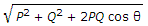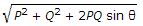# Civil Engineering - Applied Mechanics

### Exercise :: Applied Mechanics - Section 1

31.

If three rigid rods are hinged together to form a triangle and are given rotary as well as translatory motion, the number of instantaneous centres of the triangle, will be

 A. 1 B. 2 C. 3 D. 4 E. 5

Explanation:

No answer description available for this question. Let us discuss.

32.

The mechanical advantage of an ideal machine is 100. For moving the local through 2 m, the effort moves through

 A. 0.02 m B. 2 m C. 2.5 m D. 20 m.

Explanation:

No answer description available for this question. Let us discuss.

33.

When a body in equilibrium undergoes an infinitely small displacement, work imagined to be done, is known as

 A. imaginary work B. negative work C. virtual work D. none of these.

Explanation:

No answer description available for this question. Let us discuss.

34.

The resultant of two forces P and Q acting at an angle θ, is

 A. P2 + Q2 + 2P sin θ B. P2 + Q2 + 2PQ cos θ C. P2 + Q2 + 2PQ tan θ D.E.Explanation:

No answer description available for this question. Let us discuss.

35.

To attain the synchronous orbit, the launch of a satellite, is done from a place

 A. on equator B. on 30° latitude C. on 45° latitude D. on 60° latitude E. on the poles.